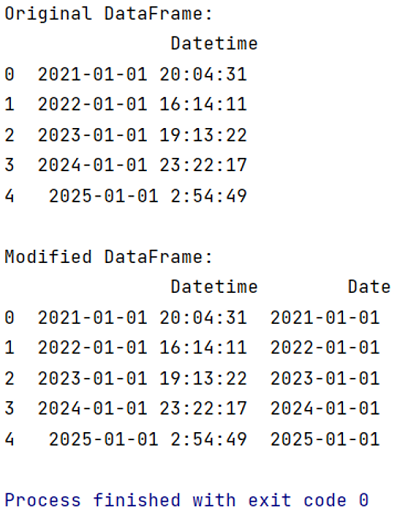# How to keep only date part when using pandas.to_datetime?

Learn how to keep only date part when using pandas.to_datetime?
Submitted by Pranit Sharma, on May 09, 2022

The datetime is a library in Python which is a collection of date and time. Inside datetime, we can access date and time in any format, but usually, the date is present in the format of "yy-mm-dd" and the time is present in the format of "HH:MM:SS’".

Here,

• yy means year
• mm means month
• dd means day
• HH means hours
• MM means minutes
• SS means seconds

While accessing the date and time from datetime, we always get date and time together, here we are going to learn how to convert Date Time to Date?

To work with pandas, we need to import pandas package first, below is the syntax:

```import pandas as pd
```

Let us understand with the help of an example:

```# Importing pandas package
import pandas as pd

# Importing datetime package
import datetime

# Creating a dictionaries of datetime
d = {'Datetime':['2021-01-01 20:04:31',
'2022-01-01 16:14:11',
'2023-01-01 19:13:22',
'2024-01-01 23:22:17',
'2025-01-01 2:54:49']}

# Creating a DataFrame
df = pd.DataFrame(d)

# Display original DataFrame
print("Original DataFrame:\n",df,"\n")

# Converting Date time to date only
# Adding a new column named Date with only dates
df['Date'] = pd.to_datetime(df['Datetime']).dt.date

# Display modified DataFrame
print("Modified DataFrame:\n",df)
```

Output:Preparation